## 目标

kaggle competitions download -c digit-recognizer


## 神经网络的推导

### M-P 神经元

• $w_i$ ，表示第 $i$ 个连进来的神经元的连接权重（weight）。
• $\theta$，表示激活阈值（threshold）。
• $f$，表示激活函数（activation function）

• $x_i$，表示第 $i$ 个连进来的神经元的输出值。
$$y=f\left(\sum_{i=1}^{n} w_{i} x_{i}-\theta\right)$$

$$y = f(\mathbf{w} \cdot \mathbf{x} - \theta )$$

### 激活函数

• Sigmoid
• Sgn
• ReLU
• Tanh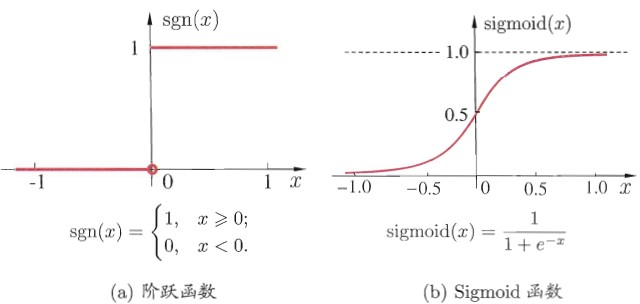### 哑结点

$$y=f\left(\sum_{i=1}^{n} w_{i} x_{i}-\theta\right) = f(\sum_{i=1}^{n+1}w_ix_i)$$

### 分层网络

• 输入层：负责直接处理输入的数据。
• 中间层：也叫隐藏层。
• 输出层：负责输出到我们限定的值域。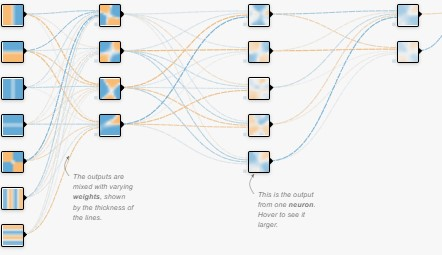### BP 算法

BP 算法是多层网络的一种训练方法。

#### 误差估计

$$C =\operatorname{MSE} = \mathbb{E}[(\hat{y} - y)^2]$$

#### 参数更新

$$v \leftarrow v + \Delta v$$

$$\Delta w_{h j}=-\eta \frac{\partial C}{\partial w}$$

• $C$ 是均方误差

• $w$ 是连到上一个结点的权重

• $\mu$ 是用来整体控制调整的快慢的。

#### 具体更新权重的公式

$$\dfrac{\partial C}{\partial w} = \dfrac{\partial C}{\partial \hat y} \cdot \dfrac{\partial \hat y}{\partial \beta} \cdot{ \color{blue}{ \dfrac{\partial \beta}{\partial w} } }$$

$\beta$ 表示神经元接收到的加权输入，$\beta = \sum_{}^{} wb = \mathbf {w} \cdot \mathbf {b}$ （$\mathbf {b}$ 表示输出值 ），即收到的各个连接的期望。因此：

$${ \color{blue}{ \dfrac{\partial \beta}{\partial w} } } = b$$

• $\dfrac{\partial C}{\partial \hat y} = \dfrac{1}{2} \cdot 1 \cdot 2(\hat{y} - y) = \hat{y} - y$
• $\hat {y} = f (\mathbf {w} \cdot \mathbf {b} - \theta ) = f (\beta - \theta$)，因此 $\dfrac {\partial \hat y}{\partial \beta} = f'(\beta - \theta) = \left{\begin {array}{cc} 1 \quad \text{when } f(\beta - \theta , 0) > 0 \ 0 \quad \text{when } f(\beta - \theta , 0) = 0 \end{array}\right.$

\begin{align} \Delta w = \eta gb \\ \Delta \theta = - \eta g \\ \Delta v = \eta ex \\ \Delta \gamma = -\eta e \end{align}

## 数字识别的实现

### 查看数据集

# example of loading the mnist dataset
from tensorflow.keras.datasets import mnist
from matplotlib import pyplot as plt
(trainX, trainy), (testX, testy) = mnist.load_data()
print('Train: X=%s, y=%s' % (trainX.shape, trainy.shape))
print('Test: X=%s, y=%s' % (testX.shape, testy.shape))
# plot first few images
for i in range(6):
# define subplot
plt.subplot(320 + 1 + i)
# plot raw pixel data
plt.imshow(trainX[i], cmap=plt.get_cmap('gray'))
# show the figure
plt.show()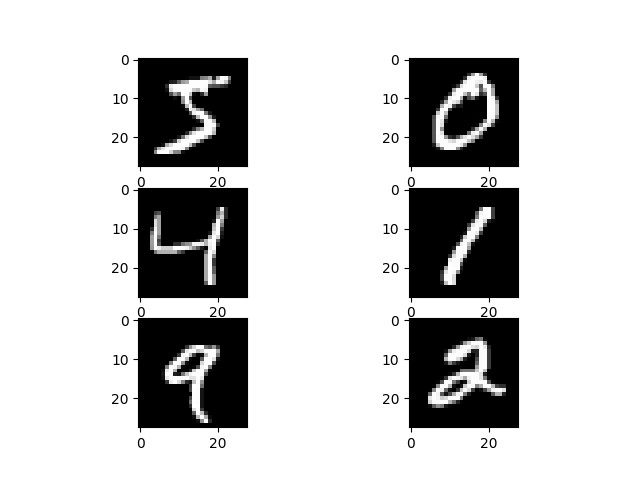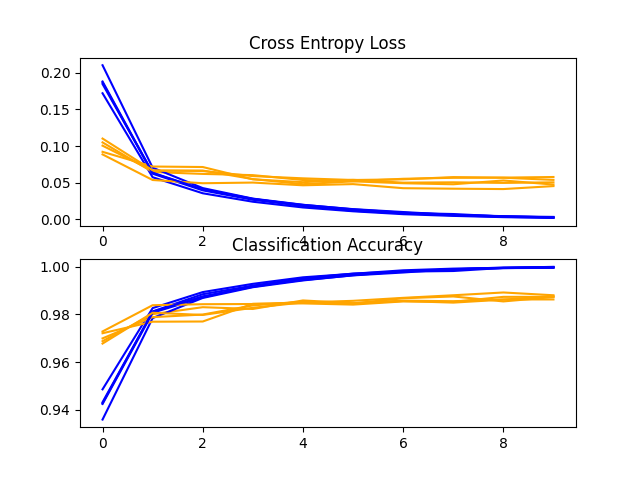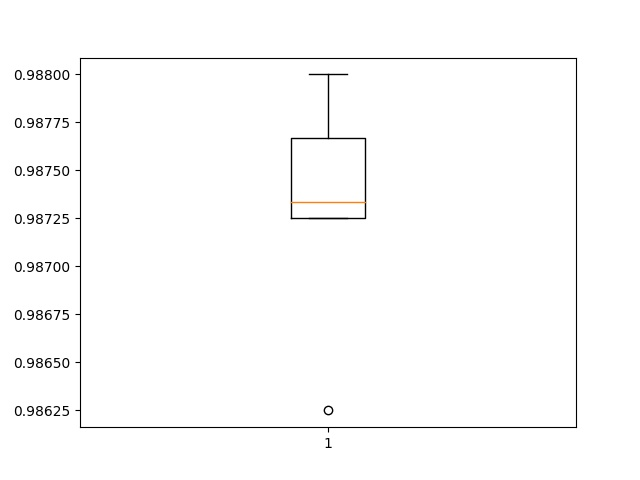> 98.625
> 98.725
> 98.733
> 98.800
> 98.767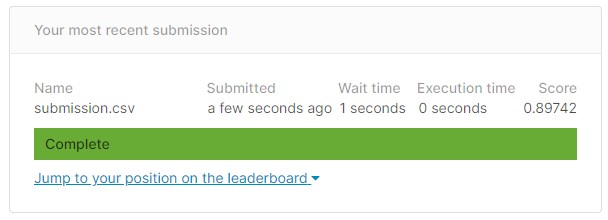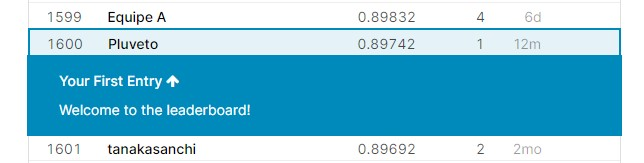Digits Recognition with CNN Keras | Kaggle

## 参考资料

Neural networks and deep learning

How to Develop a CNN for MNIST Handwritten Digit Classification (machinelearningmastery.com)